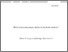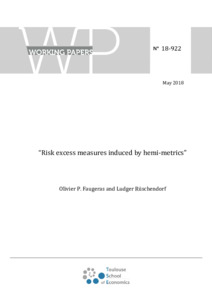Increase the visibility of your scientific production by authorizing the export of your publications to HAL!

# Risk excess measures induced by hemi-metrics

Faugeras, Olivier and Rüschendorf, Ludger (2018) Risk excess measures induced by hemi-metrics. Probability, Uncertainty and Quantitative Risk, vol. 3 (n° 1). pp. 1-35.Preview
Text

## Abstract

The main aim of this paper is to introduce the notion of risk excess measure, to analyze its properties and to describe some basic construction methods. To compare the risk excess of one distribution Q w.r.t. a given risk distribution P, we propose to apply the concept of hemi-metric on the space of probability measures. This view of risk comparison has a natural basis in the extension of orderings and hemi-metrics on the underlying space to the level of probability measures. Basic examples of these kind of extensions are induced by mass transportation and by function class induced orderings. Our view towards measuring risk excess adds to the usually considered method to compare risks of Q and P by the values rho(Q), rho(P) of a risk measure rho. We argue that the difference rho(Q)-rho(P) neglects relevant aspects of the risk excess which are adequately described by the new notion of risk excess measure. We derive various concrete classes of risk excess measures and discuss corresponding ordering and measure extension properties.

Item Type: Article English 2018 Yes B- ECONOMIE ET FINANCE TSE-R (Toulouse) UT1 02 Oct 2019 13:12 02 Oct 2019 13:12 oai:tse-fr.eu:123591 http://publications.ut-capitole.fr/id/eprint/32822

### Available Versions of this ItemView Item